Ch 5. Rigid Body General Motion Multimedia Engineering Dynamics Fixed Axis Rotation Plane Motion Velocities Zero Velocity Point Plane Motion Accelerations Multiple Gears Rot. Coord. Velocities Rot. Coord. Acceleration
 Chapter - Particle - 1. General Motion 2. Force & Accel. 3. Energy 4. Momentum - Rigid Body - 5. General Motion 6. Force & Accel. 7. Energy 8. Momentum 9. 3-D Motion 10. Vibrations Appendix Basic Math Units Basic Equations Sections Search eBooks Dynamics Fluids Math Mechanics Statics Thermodynamics Author(s): Kurt Gramoll ©Kurt GramollDYNAMICS - CASE STUDY SOLUTION This problem involves two rotating disks, which suggests using two separate coordinate systems.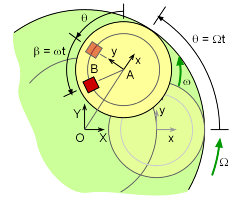Angles θ and β orientation The main disk's angular velocity, Ω, is 1 rad/s about the fixed X-Y axes. The rider disk is attached to the main disk 3 meters from the center and has an angular velocity, ω, of 2 rad/s relative to the main disk. The rotating x-y axes are attached to the main disk and rotate with the main disk. The rider is 1.5 m from the rider-disk center. The question requires the velocity at t = 2.4 s. Since both the time and angular velocities are known, the rotational position of both disks can be determined by θ = Ω t and β = ω t. The final position can be determined with θ and β known. The disks are assumed to be at full speed at t = 0.0 s. This ignores all start up accelerations (accelerations are examined in the next section). Use the relative velocity equation in the I-J fixed coordinate system (the i-j system could also be used):      vB = vA + Ω × rB/A + vB/A Analyze each term in the equation. Term vA (motion of A about center pt. O)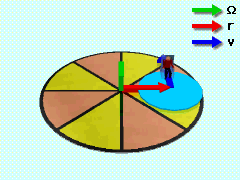Velocity of A The first and simplest term is vA.      vA = Ω × rA/O           = 1K × 3(cosθI + sinθ J)           = 3(cos(Ωt) (K×I) + 3(sin(Ωt) (K×J)           = -3 sin(1(2.4)) I + 3 cos(1(2.4)) J           = -2.03 I - 2.21J Notice that all the vectors can be described in the rotating i-j or the non-rotating I-J coordinate system. However, do not mix coordinate systems. Term Ω×rB/A (motion of B, rotating axis)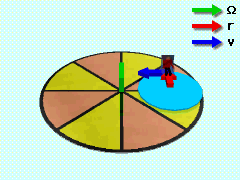Velocity of B Due to Rotation Next, look at the rotating axis term. It is important to note that the total rotated angle for the position vector, rB/A is θ + β.      Ω × rB/A = 1K × 1.5 (-sin(θ+β)I + cos(θ+β)J)          = -1.5 sin(Ωt+ωt) (K×I) + 1.5 cos(Ωt+ωt)(K×J))          = -1.5 cos(3(2.4))I - 1.5 sin(3(2.4)) J          = -0.912I - 1.190J m/s Term vB/A (motion of B with respect to A)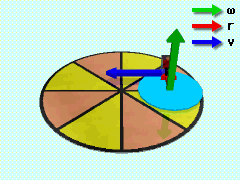Velocity of B with Respect to A vB/A = ω × rB/A           = 2K × 1.5(-sin(θ+β)I + cos(θ+β)J)           = -3 sin(3t) (K×I) + 3 cos(3t) (K×J))           = -3 cos(3 (2.4)) I - 3 sin(3 (2.4)) J           = -1.82 I - 2.38 J All Terms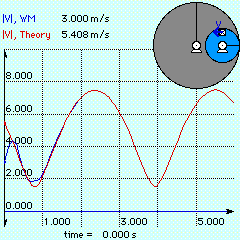Theory vs. Numerical Results Now, putting all three terms together gives      vB = (-2.03 - 0.912 -1.82)I + (-2.12 - 1.19 -2.38)J      vB = -4.76I - 5.78J The maximum occurs at about 2.4 seconds and will repeat the maximum velocity every 3 seconds. This rapid repeating of the maximum velocity makes the ride exciting. However, the maximum acceleration also needs to be be investigated to insure the ride is safe. This done in the next section.

Practice Homework and Test problems now available in the 'Eng Dynamics' mobile app
Includes over 400 problems with complete detailed solutions.
Available now at the Google Play Store and Apple App Store.